Miscellaneous

Chapter 9 Class 11 Sequences and Series (Term 1)
Serial order wise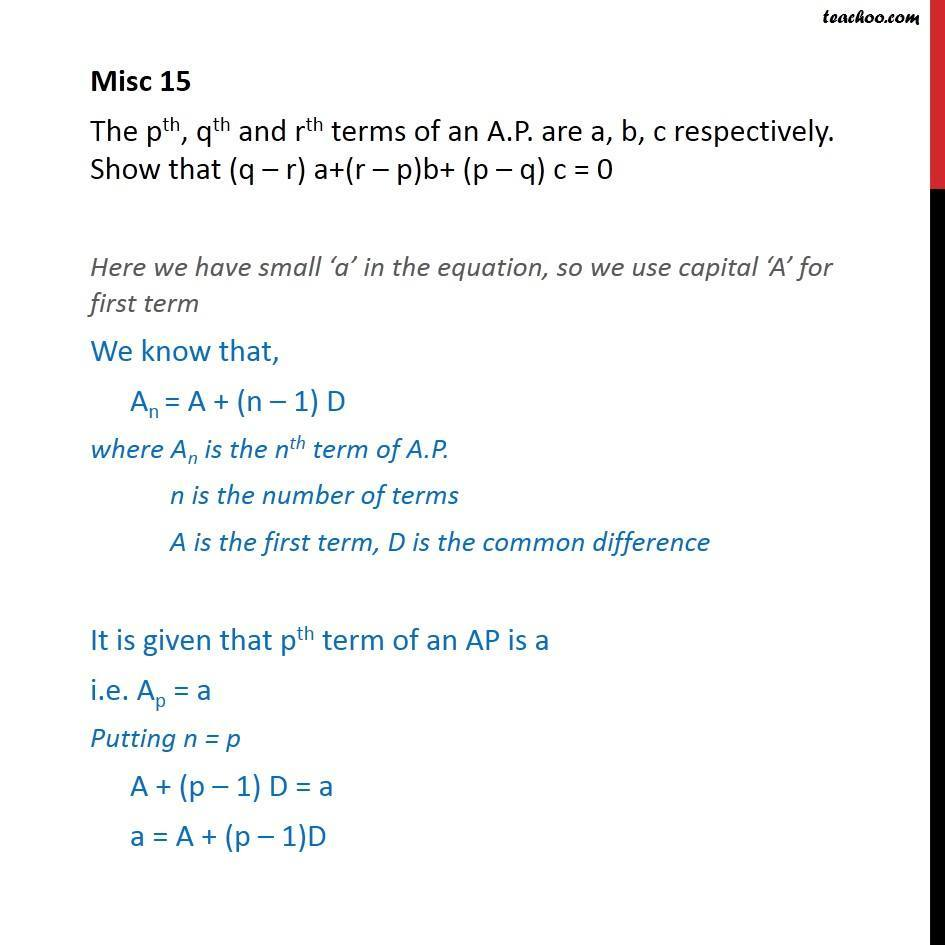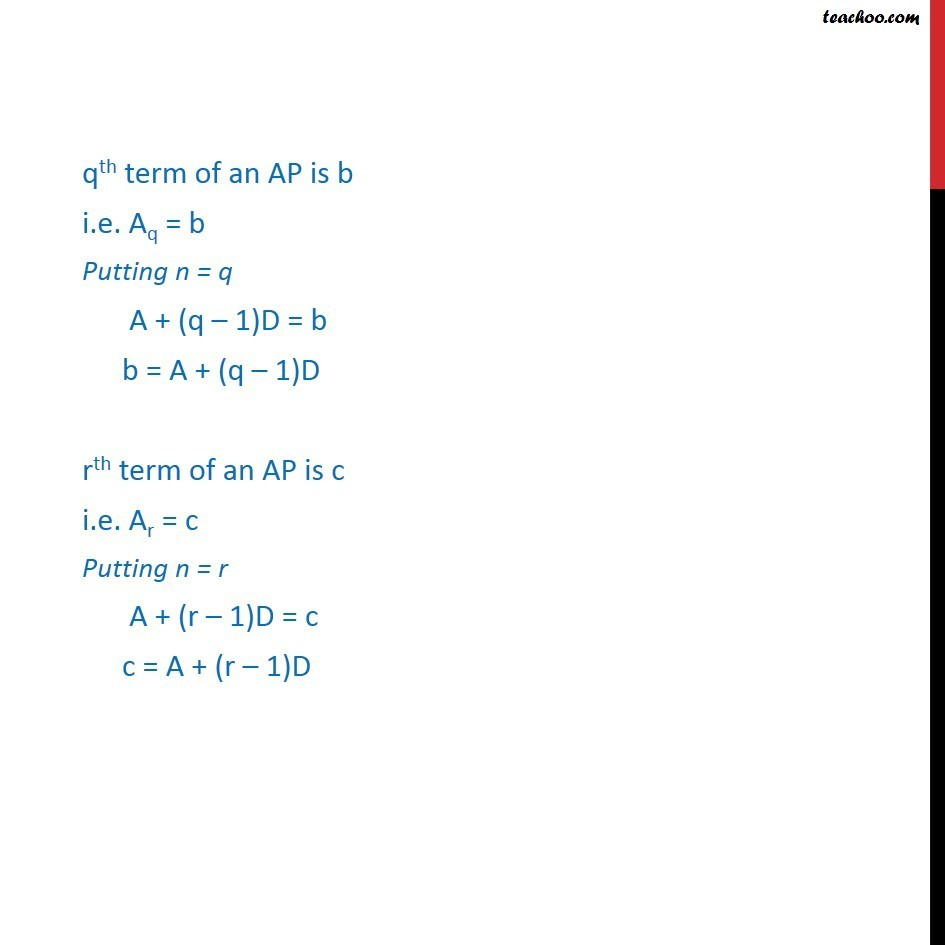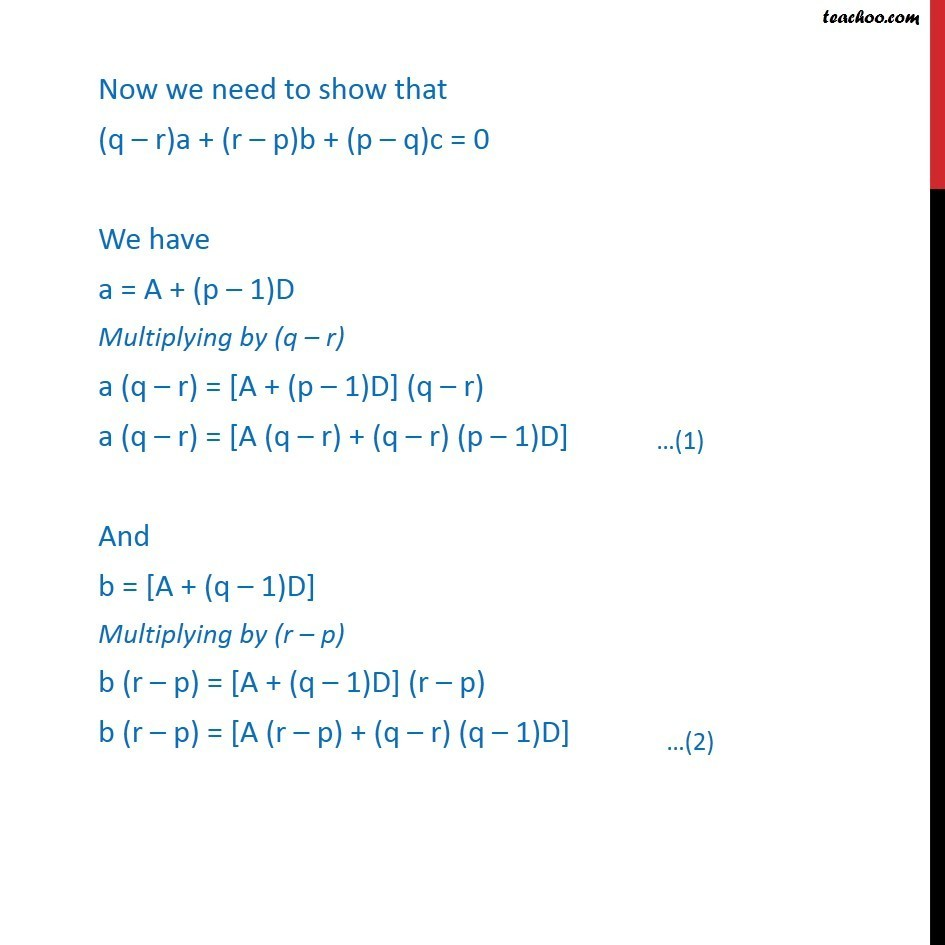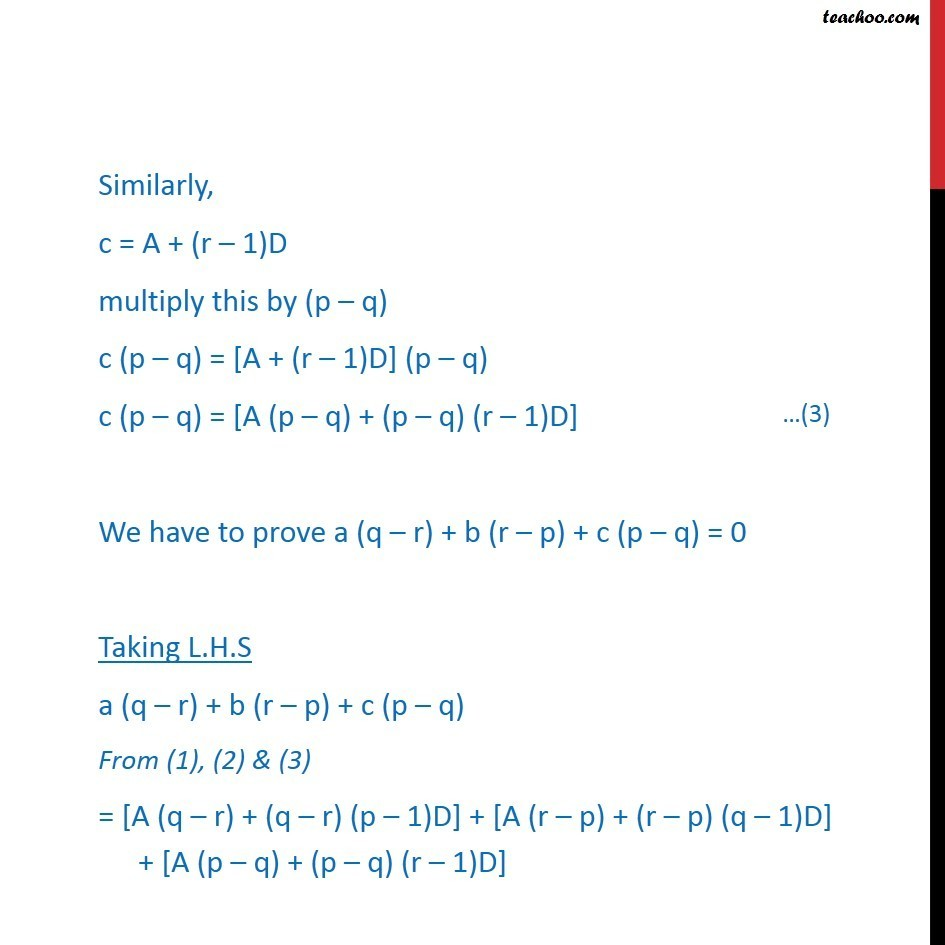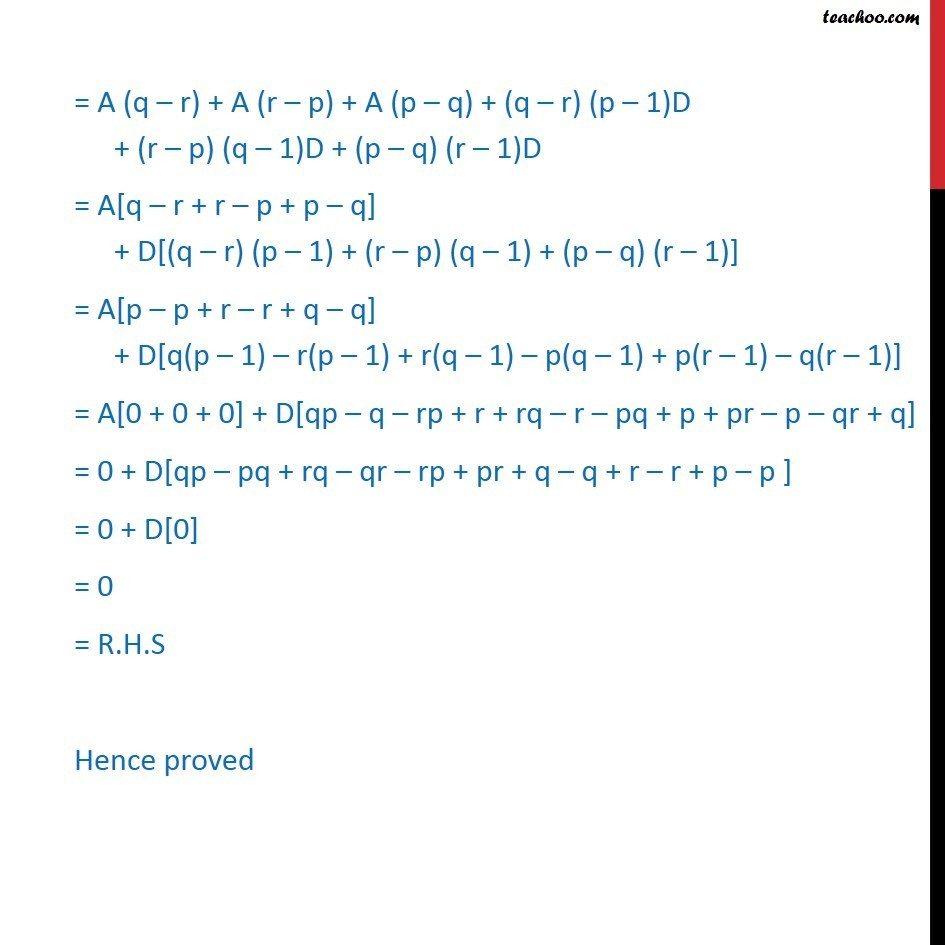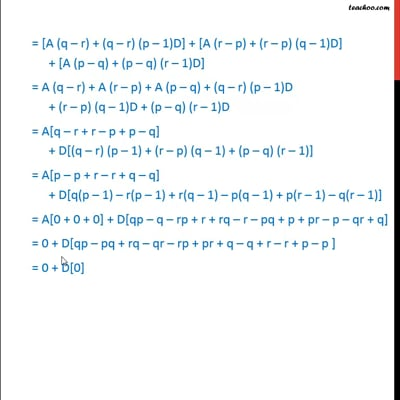This video is only available for Teachoo black users

### Transcript

Misc 15 The pth, qth and rth terms of an A.P. are a, b, c respectively. Show that (q r) a+(r p)b+ (p q) c = 0 Here we have small a in the equation, so we use capital A for first term We know that, An = A + (n 1) D where An is the nth term of A.P. n is the number of terms A is the first term, D is the common difference It is given that pth term of an AP is a i.e. Ap = a Putting n = p A + (p 1) D = a a = A + (p 1)D qth term of an AP is b i.e. Aq = b Putting n = q A + (q 1)D = b b = A + (q 1)D rth term of an AP is c i.e. Ar = c Putting n = r A + (r 1)D = c c = A + (r 1)D Now we need to show that (q r)a + (r p)b + (p q)c = 0 We have a = A + (p 1)D Multiplying by (q r) a (q r) = [A + (p 1)D] (q r) a (q r) = [A (q r) + (q r) (p 1)D] And b = [A + (q 1)D] Multiplying by (r p) b (r p) = [A + (q 1)D] (r p) b (r p) = [A (r p) + (q r) (q 1)D] Similarly, c = A + (r 1)D multiply this by (p q) c (p q) = [A + (r 1)D] (p q) c (p q) = [A (p q) + (p q) (r 1)D] We have to prove a (q r) + b (r p) + c (p q) = 0 Taking L.H.S a (q r) + b (r p) + c (p q) From (1), (2) & (3) = "[A (q r) + (q r) (p 1)D] + [A (r p) + (r p) (q 1)D]" "+ [A (p q) + (p q) (r 1)D]" = "A (q r) + A (r p) + A (p q) + (q r) (p 1)D" "+ (r p) (q 1)D + (p q) (r 1)D" = A[q r + r p + p q] + D[(q r) (p 1) + (r p) (q 1) + (p q) (r 1)] = A[p p + r r + q q] + D[q(p 1) r(p 1) + r(q 1) p(q 1) + p(r 1) q(r 1)] = A[0 + 0 + 0] + D[qp q rp + r + rq r pq + p + pr p qr + q] = 0 + D[qp pq + rq qr rp + pr + q q + r r + p p ] = 0 + D = 0 = R.H.S Hence proved